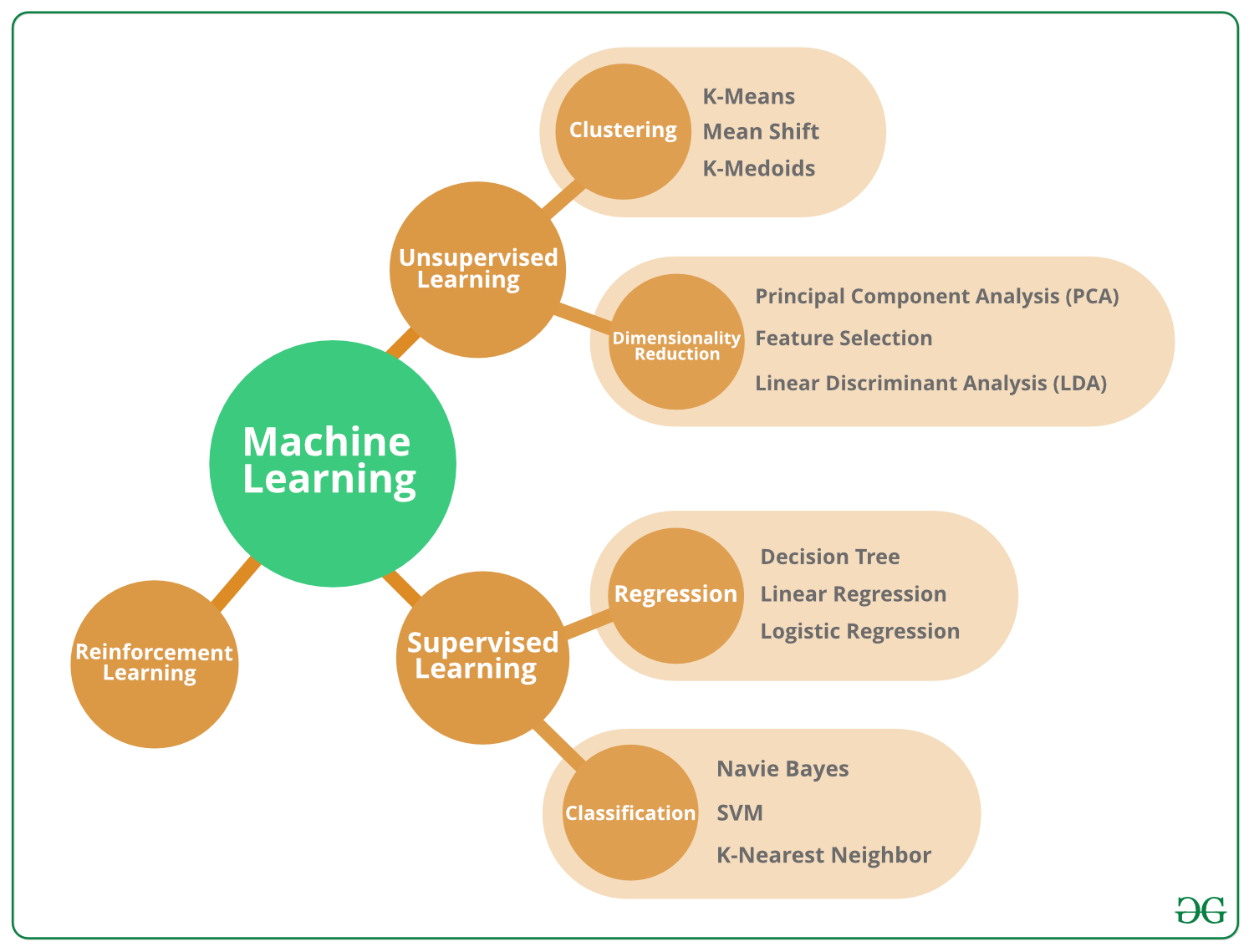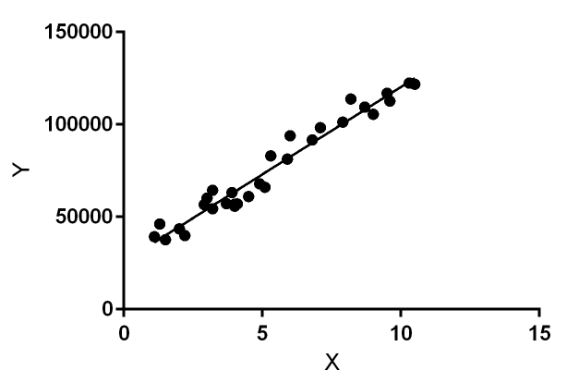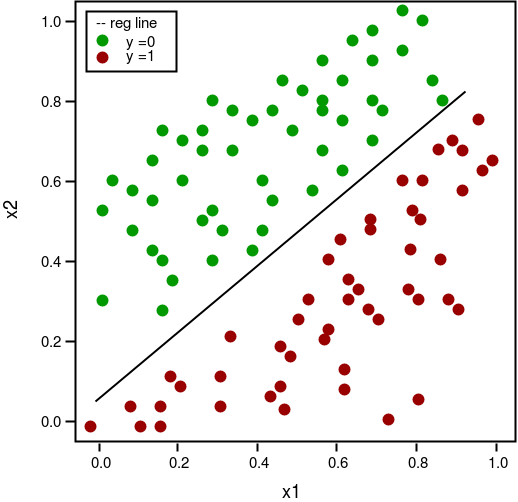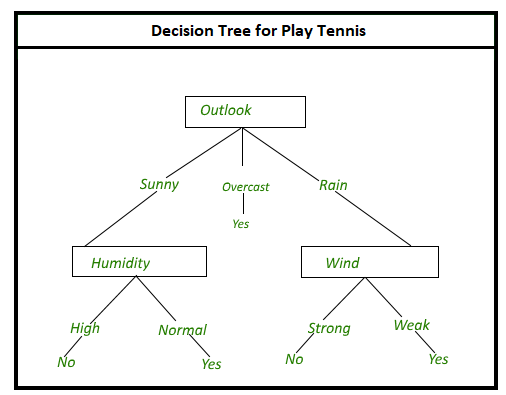Related Articles
Top 10 Algorithms every Machine Learning Engineer should know
• Difficulty Level : Hard
• Last Updated : 20 Aug, 2019

Computers are able to see, hear and learn. Welcome to the future.

And Machine Learning is the future. According to Forbes, Machine learning patents grew at a 34% Rate between 2013 and 2017 and this is only set to increase in coming times. Moreover, a Harvard Business review article called a Data Scientist as the “Sexiest Job of the 21st Century” (And that’s incentive right there!!!).

In these highly dynamic times, there are various machine learning algorithms developed to solve complex real-world problems. These algorithms are highly automated and self-modifying as they continue to improve over time with the addition of an increased amount of data and with minimum human intervention required. So this article deals with the Top 10 Machine Learning algorithms.

But to understand these algorithms, first, the different types they can belong to are explained briefly.### Types of Machine Learning Algorithms –

Machine Learning algorithms can be classified into 3 different types, namely:

Supervised Machine Learning Algorithms:
Imagine a teacher supervising a class. The teacher already knows the correct answers but the learning process doesn’t stop until the students learn the answers as well (poor kids!). This is the essence of Supervised Machine Learning Algorithms. Here, the algorithm is the student that learns from a training dataset and makes predictions that are corrected by the teacher. This learning process continues until the algorithm achieves the required level of performance.

Unsupervised Machine Learning Algorithms:
In this case, there is no teacher for the class and the poor students are left to learn for themselves! This means that for Unsupervised Machine Learning Algorithms, there is no specific answer to be learned and there is no teacher. The algorithm is left unsupervised to find the underlying structure in the data in order to learn more and more about the data itself.

Reinforcement Machine Learning Algorithms:
Well, here are hypothetical students learn from their own mistakes over time (that’s like life!). So the Reinforcement Machine Learning Algorithms learn optimal actions through trial and error. This means that the algorithm decides the next action by learning behaviors that are based on its current state and that will maximize the reward in the future.

### Top Machine Learning Algorithms

There are specific machine learning algorithms that were developed to handle complex real-world data problems. So, now that we have seen the types of machine learning algorithms, let’s study the top machine learning algorithms that exist and are actually used by data scientists.

1. Naïve Bayes Classifier Algorithm –
What would happen if you had to classify data texts such as a web page, a document or an email manually? Well, you would go mad! But thankfully this task is performed by the Naïve Bayes Classifier Algorithm. This algorithm is based on the Bayes Theorem of Probability(you probably read that in maths) and it allocates the element value to a population from one of the categories that are available.where, y is class variable and X is a dependent feature vector (of size n) where:An example of the Naïve Bayes Classifier Algorithm usage is for Email Spam Filtering. Gmail uses this algorithm to classify an email as Spam or Not Spam.

2. K Means Clustering Algorithm –
Let’s imagine that you want to search the term “date” on Wikipedia. Now, “date” can refer to a fruit, a particular day or even a romantic evening with your love!!! So Wikipedia groups the web pages that talk about the same ideas using the K Means Clustering Algorithm (since it is a popular algorithm for cluster analysis).

K Means Clustering Algorithm in general uses K number of clusters to operate on a given data set. In this manner, the output contains K clusters with the input data partitioned among the clusters(As pages with different “date” meanings were partitioned).

3. Support Vector Machine Algorithm –
The Support Vector Machine Algorithm is used for classification or regression problems. In this, the data is divided into different classes by finding a particular line (hyperplane) which separates the data set into multiple classes. The Support Vector Machine Algorithm tries to find the hyperplane that maximizes the distance between the classes (known as margin maximization) as this increases the probability of classifying the data more accurately.

An example of the Support Vector Machine Algorithm usage is for comparison of stock performance for stocks in the same sector. This helps in managing investment making decisions by the financial institutions.

4. Apriori Algorithm –
The Apriori Algorithm generates association rules using the IF_THEN format. This means that IF event A occurs, then event B also occurs with a certain probability. For example: IF a person buys a car, THEN they also buy car insurance. The Apriori Algorithm generates this association rule by observing the number of people who bought car insurance after buying a car.

An example of the Apriori Algorithm usage is for Google auto-complete. When a word is typed in Google, the Apriori Algorithm looks for the associated words that are usually typed after that word and displays the possibilities.

5. Linear Regression Algorithm –
The Linear Regression Algorithm shows the relationship between an independent and a dependent variable. It demonstrates the impact on the dependent variable when the independent variable is changed in any way. So the independent variable is called the explanatory variable and the dependent variable is called the factor of interest.An example of the Linear Regression Algorithm usage is for risk assessment in the insurance domain. Linear Regression analysis can be used to find the number of claims for customers of multiple ages and then deduce the increased risk as to the age of the customer increases.

6. Logistic Regression Algorithm –
The Logistic Regression Algorithm deals in discrete values whereas the Linear Regression Algorithm handles predictions in continuous values. So, Logistic Regression is suited for binary classification wherein if an event occurs, it is classified as 1 and if not, it is classified as 0. Hence, the probability of a particular event occurrence is predicted based on the given predictor variables.An example of the Logistic Regression Algorithm usage is in politics to predict if a particular candidate will win or lose a political election.

7. Decision Trees Algorithm –
Suppose that you want to decide the venue for your birthday. So there are many questions that factor in your decision such as “Is the restaurant Italian?”, “Does the restaurant have live music?”, “Is the restaurant close to your house?” etc. Each of these questions has a YES or NO answer that contributes to your decision.

This is what basically happens in the Decision Trees Algorithm. Here all possible outcomes of a decision are shown using a tree branching methodology. The internal nodes are tests on various attributes, the branches of the tree are the outcomes of the tests and the leaf nodes are the decision made after computing all of the attributes.An example of the Decision Trees Algorithm usage is in the banking industry to classify loan applicants by their probability of defaulting said loan payments.

8. Random Forests Algorithm –
The Random Forests Algorithm handles some of the limitations of Decision Trees Algorithm, namely that the accuracy of the outcome decreases when the number of decisions in the tree increases.

So, in the Random Forests Algorithm, there are multiple decision trees that represent various statistical probabilities. All of these trees are mapped to a single tree known as the CART model. (Classification and Regression Trees). In the end, the final prediction for the Random Forests Algorithm is obtained by polling the results of all the decision trees.

An example of the Random Forests Algorithm usage is in the automobile industry to predict the future breakdown of any particular automobile part.

9. K Nearest Neighbours Algorithm –
The K Nearest Neighbours Algorithm divides the data points into different classes based on a similar measure such as the distance function. Then a prediction is made for a new data point by searching through the entire data set for the K most similar instances (the neighbors) and summarizing the output variable for these K instances. For regression problems, this might be the mean of the outcomes and for classification problems, this might be the mode (most frequent class).

The K Nearest Neighbours Algorithm can require a lot of memory or space to store all of the data, but only performs a calculation (or learns) when a prediction is needed, just in time.

10. Artificial Neural Networks Algorithm –
The human brain contains neurons that are the basis of our retentive power and sharp wit(At least for some of us!) So the Artificial Neural Networks try to replicate the neurons in the human brain by creating nodes that are interconnected to each other. These neurons take in information through another neuron, perform various actions as required and then transfer the information to another neuron as output.

An example of Artificial Neural Networks is Human facial recognition. Images with human faces can be identified and differentiated from “non-facial” images. However, this could take multiple hours depending on the number of images in the database whereas the human mind can do this instantly.My Personal Notes arrow_drop_up